# Paper Walkthrough — Matrix Calculus for Deep Learning (Part 2 / 2)

1. The Vector Chain Rule.
2. The gradient of the neuron activation function.

# The Vector Chain Rule

While I assume that you already aware of the chain rule for differentiation, the vector chain rule deserves VIP status.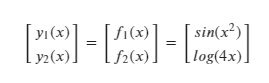Eq.1 : Vector function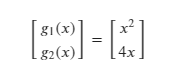Eq.2 : Variables used for substitution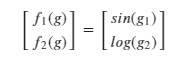Eq.3 : Substituted new variables in the Vector function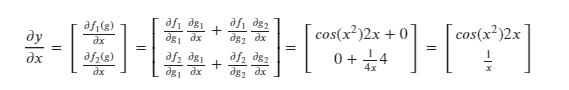Eq.4 : Vector chain rule for differentiation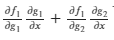Eq.5 : Chain rule applied to the first vector function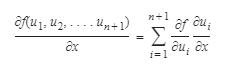Eq.6 : Generalized vector chain rule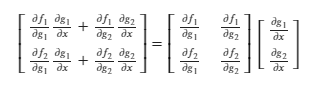Eq.7 : Vector chain rule as product of Jacobians

# The gradient of neuron activation

We can now find the derivative of-

# The gradient of the neural network loss function

Say we have the following inputs to our neural network: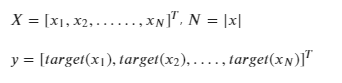Eq.20 : Inputs and targets to the neural network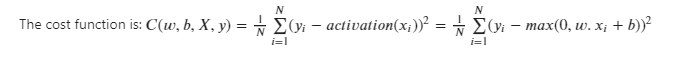Eq.21 : Cost function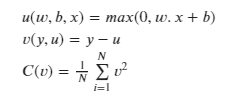Eq.22 : Intermediate variables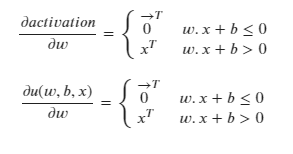Eq.23 : Derivative of the activation function with respect to the weights and the biases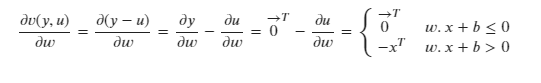Eq.24 : Derivative of v with respect to the weights.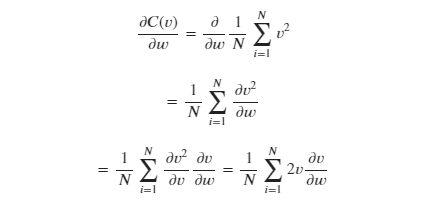Eq.25 : Differentiating C with respect to w.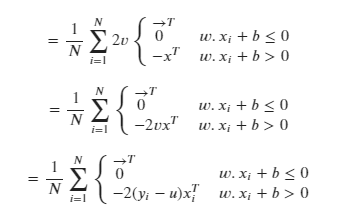Eq.26 : Substituting for the partial derivative of v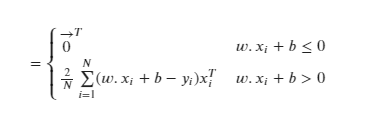Eq.27 : Derivative of the cost function with respect to the weights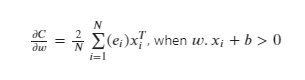Eq.28 : Substituting the difference between the actual and target values as the error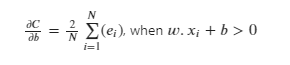Eq.29 : Derivative of the cost function with respect to the bias

--

--## Kaushik Moudgalya

Computer Science Master’s student at the University of Montreal, specializing in Machine Learning.